# Reflection Of Light

##### Class 9th Science & Technology MHB Solution
Exercises
1. Explain the difference between a plane mirror, a concave mirror and a convex mirror with…
2. Describe the positions of the source of light with respect to a concave mirror in 1. Torch…
3. Why are concave mirrors used in solar devices? Answer the following questions.…
4. Why are the mirrors fitted on the outside of cars convex? Answer the following questions.…
5. Why does obtaining the image of the sun on a paper with the help of a concave mirror burn…
6. If a spherical mirror breaks, what type of mirrors are the individual pieces? Answer the…
7. What sign conventions are used for reflection from a spherical mirror?…
8. Draw ray diagrams for the cases of images obtained in concave mirrors as described in the…
9. Which type of mirrors are used in the following? Periscope, floodlights, shaving mirror,…
10. An object of height 7 cm is kept at a distance of 25 cm in front of a concave mirror. The…
11. A convex mirror has a focal length of 18 cm. The image of an object kept in front of the…
12. A 10 cm long stick is kept in front of a concave mirror having focal length of 10 cm in…
13. Three mirrors are created from a single sphere. Which of the following - pole, center of…

###### Exercises
Question 1.

Explain the difference between a plane mirror, a concave mirror and a convex mirror with respect to the type and size of the images produced.

Plane Mirror: The image formed by plane mirror is Virtual (can’t be taken on the screen), Upright. And size of image is same as the size of the object. Also distance between object and mirror is same as the distance between mirror and image.

Concave Mirror: The image formed by concave mirror is both

real(can be taken on screen) and virtual. If image is real than it is

inverted and is found in front of mirror. If image is virtual than

image is upright and is formed behind the mirror. The size of

image can be smaller and larger than the object size depending on

the position of the object.

Convex Mirror: The image formed by convex mirror is always

virtual, upright and smaller than size of the object.

Question 2.

Describe the positions of the source of light with respect to a concave mirror in

1. Torch light .

2. Projector lamp.

3. Floodlight.

1.) Torch Light : The Source of light is placed at the Focus of the Concave Mirror because in Concave Mirror if the object is placed at Focus than its image is formed at the infinity hence we can get a light to longer distances .

2.) Projector Lamp: The source of light is placed at the Center Of Curvature because if object is placed at the center of curvature than its image is same as the size of the object, real. Hence image can be taken on the screen Like Whiteboard etc due to its real nature and size will also be sufficient not too large and too small.

3.) Flood Light: The Source of light is placed Beyond The Centre Of Curvature because the image formed is real and Magnified. hence we can get wider area of light.

Question 3.

Why are concave mirrors used in solar devices?

Concave Mirrors are used in the solar devices like Solar Cooker, Solar Furnace etc. because they are the only mirror which reflects the light towards the focus (Plane mirrors don’t have focus, convex mirrors have imaginary focus behind the mirror which reflects light away from the focus ).

The light collected at the Focus has lot of thermal energy which can be used in many ways.

Question 4.

Why are the mirrors fitted on the outside of cars convex?

Mirrors fitted outside of cars are convex because convex mirrors always formed virtual and very diminished image. Hence we can see large traffic in a smaller area of the mirror.

Question 5.

Why does obtaining the image of the sun on a paper with the help of a concave mirror burn the paper?

The paper is burnt because the rays of the sun which comes from infinite distance forms the image at the focus of the concave mirror hence large amount of heat is generated due to infinite rays of sun meeting at the focus which in turn burns the paper.

Question 6.

If a spherical mirror breaks, what type of mirrors are the individual pieces?

The individual pieces of the broken spherical mirror are the spherical mirror itself. It may be of two types:

a.) Concave (reflecting surface curved inwards).

b.)Convex (reflecting surface curved outwards).

Question 7.

What sign conventions are used for reflection from a spherical mirror?

Pole (P) of the mirror is taken as the origin and the Principal axis is taken as the horizontal X- axis. The sign conventions are as follows:

1. The object is always placed on the left side of the mirror.

2. All the distances measured to the right side of the origin are taken as positive, while the distances measured to the left side of the origin are taken as negative.

3. All the distances parallel to the principal axis of the mirror are measured from the pole of the mirror.

4. Distances measured vertically upwards from the principal axis of the mirror are taken as positive.

5. Distances measured vertically downwards from the principal axis of the mirror are taken as negative.

6. The focal length of Concave mirror is negative while that of convex mirror is positive.

Question 8.

Draw ray diagrams for the cases of images obtained in concave mirrors as described in the table on page 122.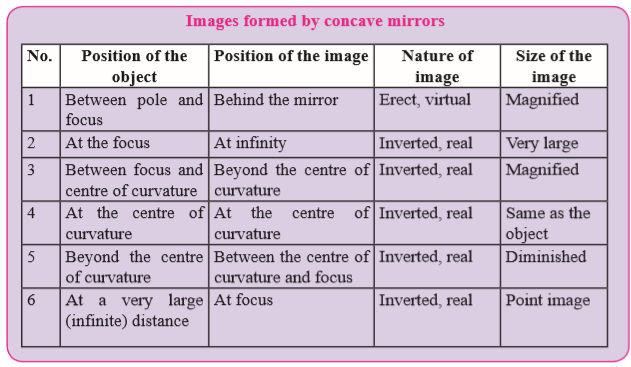1. When Object is between pole and focus.

Ø Position of Image => Behind the mirror.

Ø Nature of Image => Erect and Virtual.

Ø Size of Image => Magnified.

Ø Diagram =>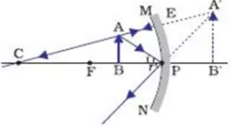2. When Object is at focus.

Ø Position of Image => At Infinity

Ø Nature of Image => Real and Inverted.

Ø Size of Image => Very Large.

Ø Diagram =>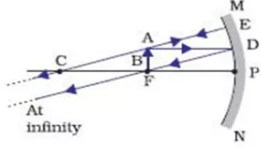3. When Object is between focus and center of curvature.

Ø Position of Image =>Beyond the center of curvature.

Ø Nature of Image => Inverted and real.

Ø Size of Image => Magnified.

Ø Diagram =>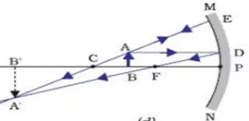4. When Object is at center of curvature.

Ø Position of Image =>At center of curvature.

Ø Nature of Image => Inverted and real.

Ø Size of Image => Same as Object.

Ø Diagram =>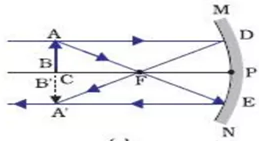5. When Object is beyond center of curvature.

Ø Position of Image =>Between center of curvature and focus.

Ø Nature of Image => Inverted and real.

Ø Size of Image => Diminished.

Ø Diagram =>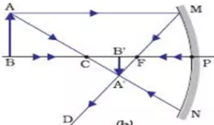6. When Object is at very large distance(Infinity).

Ø Position of Image => At focus.

Ø Nature of Image => Inverted and real.

Ø Size of Image => Point size.

Ø Diagram =>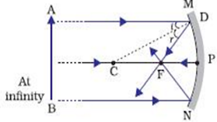Question 9.

Which type of mirrors are used in the following?

Periscope, floodlights, shaving mirror, kaleidoscope, street lights, head lamps of a car.

1.) Periscope: Simple Periscope uses Plane Mirrors because light just strikes the mirror to reach viewer eye.

2.) Flood Lights: Concave Mirrors are used in the floodlights.

3.) Shaving Mirror: Concave Mirrors are used in shaving mirrors because when the face is kept between the focus and pole of the mirror the magnified, erect and virtual image is formed.

4.) Kaleidoscope: Plane Mirrors are used in the Kaleidoscope because only reflecting surface is needed.

5.) Street Light: Convex Mirrors are used in the street light because it diverges the rays of the light upto large distance .

6.) Head Lamp of car: Concave mirrors are used in the Head Lamp of car because if the bulb is kept at focus of the mirror the rays from bulb will go to a larger distances ( infinity).

Question 10.

Solve the following examples

An object of height 7 cm is kept at a distance of 25 cm in front of a concave mirror. The focal length of the mirror is 15 cm. At what distance from the mirror should a screen be kept so as to get a clear image? What will be the size and nature of the image?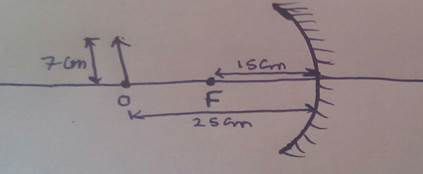According to the question,

Object distance (u) = -25 cm;

Height of object (Ho) = + 7 cm;

Focal length (f) = -15 cm;

Image distance = v cm;

By Mirror Formula: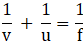1/v + 1/u = 1/f.

Putting values we get,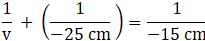⇒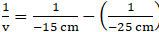⇒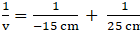Taking the lcm, we get,

⇒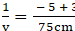⇒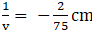⇒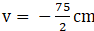⇒ v = -37.5 cm

∴ the image distance = 37.5 cm (The negative sign indicates that the image is formed on the left side of the mirror)

Now, image distance (v) = -37.5 cm

Magnification =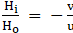Hi is the image height.

HO is the object height.

“v” is the image distance

“u” is the object distance

⇒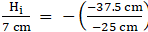⇒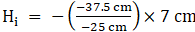⇒ Hi = -10.5 cm.

Size of image is 10.5 cm which is negative hence image is real .

Question 11.

Solve the following examples

A convex mirror has a focal length of 18 cm. The image of an object kept in front of the mirror is half the height of the object. What is the distance of the object from the mirror?

According to the question;

Focal length (f) = + 18cm;

Height of Image = Hi ;

Height of object = Ho ;

Given That;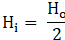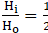= Magnification;

We Know that

Magnification =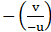=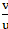(Since object is always kept to left side of mirror hence u is negative)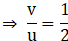Hence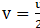By Mirror Formula;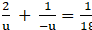Since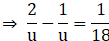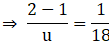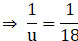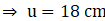.

Hence distance of the object from mirror is 18 cm.

Question 12.

Solve the following examples

A 10 cm long stick is kept in front of a concave mirror having focal length of 10 cm in such a way that the end of the stick closest to the pole is at a distance of 20 cm. What will be the length of the image?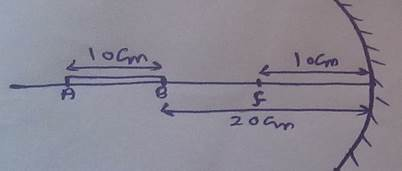According to the question;

Focal length (f) = -10cm;

Length of stick (Lo) = 10cm;

Length of image = Li;

Object distance (u) = -20cm;

By mirror formula;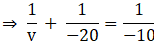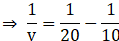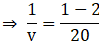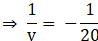⇒ v = -20.

Since v = - 20 cm which is negative hence image is at left side of mirror.

Now

Magnification =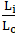=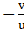Putting values of v and u .

Magnification =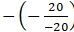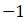Negative sign means image is inverted.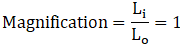Hence Li = Lo .

Hence length of image is 10 cm

Question 13.

Three mirrors are created from a single sphere. Which of the following - pole, center of curvature, radius of curvature, principal axis - will be common to them and which will not be common?

1.) Radius of Curvature = It will be common because the radius of the sphere of which the mirror is a part will be same for every part of the mirror.

2.) Principal Axis = It will not be common.

3.) Pole = It will not be common.

4.) Center of curvature = It will be common because the center of the sphere of which the mirror is a part will be same for every part of the mirror.

## PDF FILE TO YOUR EMAIL IMMEDIATELY PURCHASE NOTES & PAPER SOLUTION. @ Rs. 50/- each (GST extra)

SUBJECTS

HINDI ENTIRE PAPER SOLUTION

MARATHI PAPER SOLUTION
SSC MATHS I PAPER SOLUTION
SSC MATHS II PAPER SOLUTION
SSC SCIENCE I PAPER SOLUTION
SSC SCIENCE II PAPER SOLUTION
SSC ENGLISH PAPER SOLUTION
SSC & HSC ENGLISH WRITING SKILL
HSC ACCOUNTS NOTES
HSC OCM NOTES
HSC ECONOMICS NOTES
HSC SECRETARIAL PRACTICE NOTES

2019 Board Paper Solution

HSC ENGLISH SET A 2019 21st February, 2019

HSC ENGLISH SET B 2019 21st February, 2019

HSC ENGLISH SET C 2019 21st February, 2019

HSC ENGLISH SET D 2019 21st February, 2019

SECRETARIAL PRACTICE (S.P) 2019 25th February, 2019

HSC XII PHYSICS 2019 25th February, 2019

CHEMISTRY XII HSC SOLUTION 27th, February, 2019

OCM PAPER SOLUTION 2019 27th, February, 2019

HSC MATHS PAPER SOLUTION COMMERCE, 2nd March, 2019

HSC MATHS PAPER SOLUTION SCIENCE 2nd, March, 2019

SSC ENGLISH STD 10 5TH MARCH, 2019.

HSC XII ACCOUNTS 2019 6th March, 2019

HSC XII BIOLOGY 2019 6TH March, 2019

HSC XII ECONOMICS 9Th March 2019

SSC Maths I March 2019 Solution 10th Standard11th, March, 2019

SSC MATHS II MARCH 2019 SOLUTION 10TH STD.13th March, 2019

SSC SCIENCE I MARCH 2019 SOLUTION 10TH STD. 15th March, 2019.

SSC SCIENCE II MARCH 2019 SOLUTION 10TH STD. 18th March, 2019.

SSC SOCIAL SCIENCE I MARCH 2019 SOLUTION20th March, 2019

SSC SOCIAL SCIENCE II MARCH 2019 SOLUTION, 22nd March, 2019

XII CBSE - BOARD - MARCH - 2019 ENGLISH - QP + SOLUTIONS, 2nd March, 2019

HSC Maharashtra Board Papers 2020

(Std 12th English Medium)

HSC ECONOMICS MARCH 2020

HSC OCM MARCH 2020

HSC ACCOUNTS MARCH 2020

HSC S.P. MARCH 2020

HSC ENGLISH MARCH 2020

HSC HINDI MARCH 2020

HSC MARATHI MARCH 2020

HSC MATHS MARCH 2020

SSC Maharashtra Board Papers 2020

(Std 10th English Medium)

English MARCH 2020

HindI MARCH 2020

Hindi (Composite) MARCH 2020

Marathi MARCH 2020

Mathematics (Paper 1) MARCH 2020

Mathematics (Paper 2) MARCH 2020

Sanskrit MARCH 2020

Important-formula

THANKS